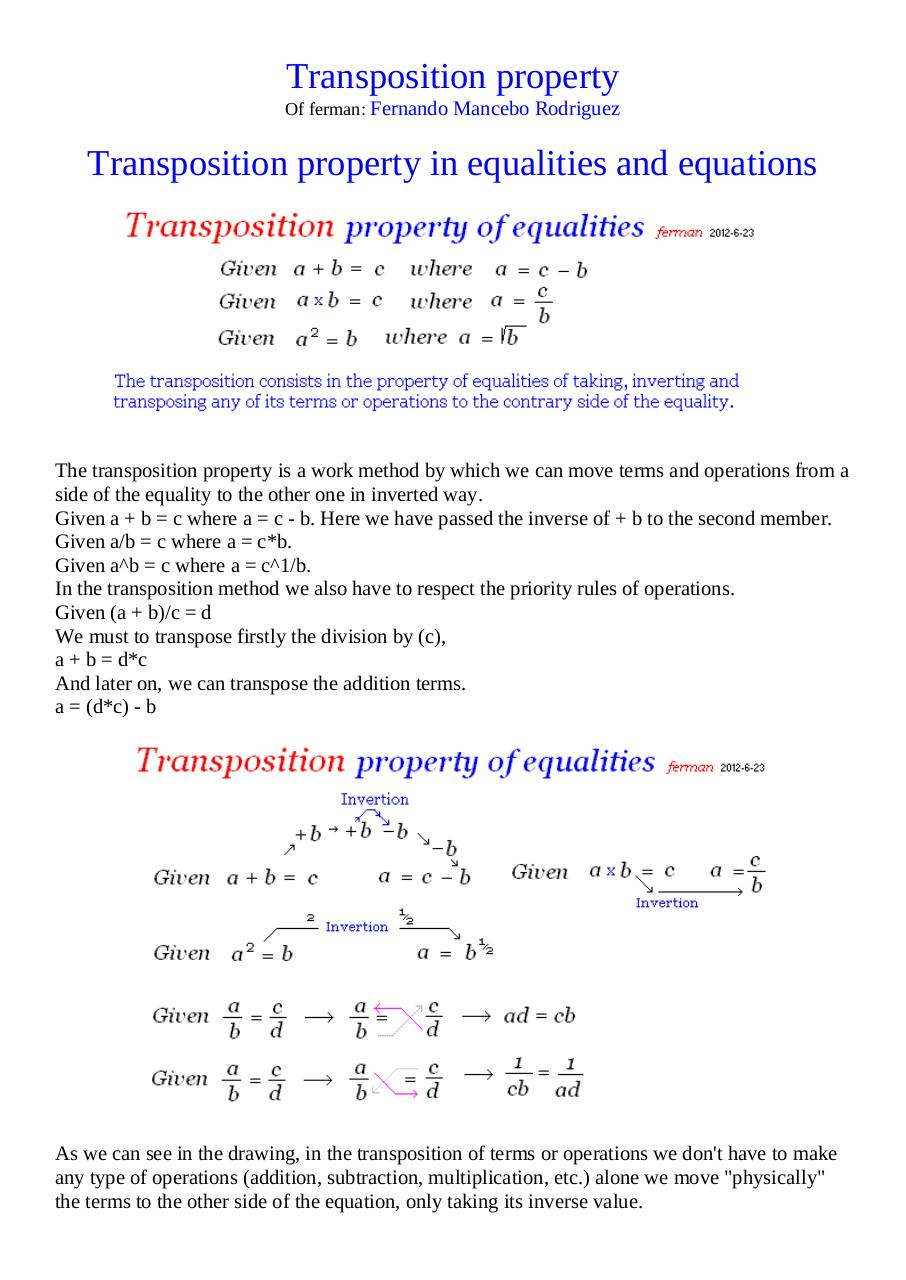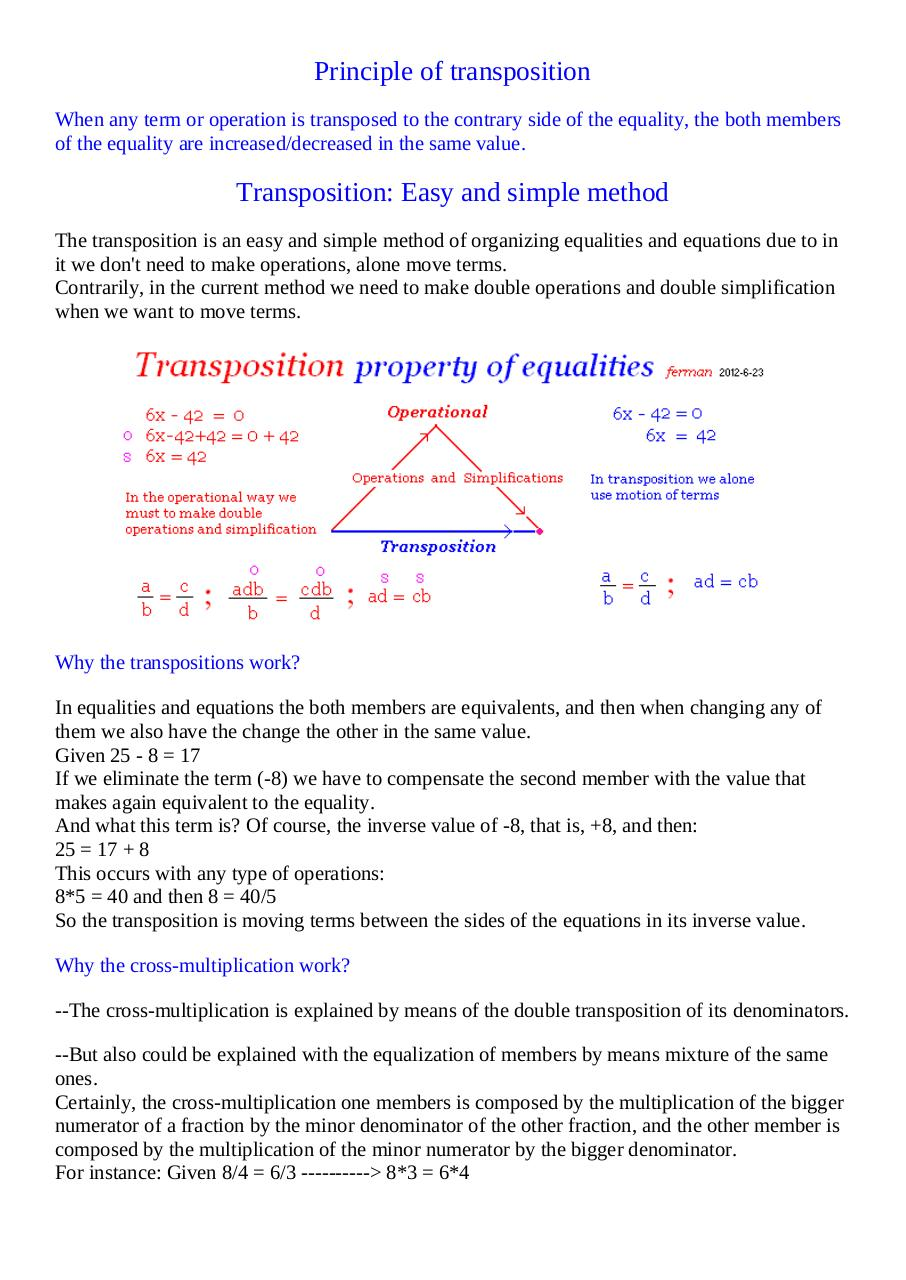# Transposition property .pdf

### File information

Original filename: Transposition property.pdf
Author: equipo

This PDF 1.5 document has been generated by Microsoft® Word 2010, and has been sent on pdf-archive.com on 14/10/2016 at 07:50, from IP address 90.168.x.x. The current document download page has been viewed 256 times.
File size: 104 KB (2 pages).
Privacy: public file

Transposition property.pdf (PDF, 104 KB)

### Document preview

Transposition property
Of ferman: Fernando Mancebo Rodriguez

Transposition property in equalities and equations

The transposition property is a work method by which we can move terms and operations from a
side of the equality to the other one in inverted way.
Given a + b = c where a = c - b. Here we have passed the inverse of + b to the second member.
Given a/b = c where a = c*b.
Given a^b = c where a = c^1/b.
In the transposition method we also have to respect the priority rules of operations.
Given (a + b)/c = d
We must to transpose firstly the division by (c),
a + b = d*c
And later on, we can transpose the addition terms.
a = (d*c) - b

As we can see in the drawing, in the transposition of terms or operations we don't have to make
any type of operations (addition, subtraction, multiplication, etc.) alone we move "physically"
the terms to the other side of the equation, only taking its inverse value.

Principle of transposition
When any term or operation is transposed to the contrary side of the equality, the both members
of the equality are increased/decreased in the same value.

Transposition: Easy and simple method
The transposition is an easy and simple method of organizing equalities and equations due to in
it we don't need to make operations, alone move terms.
Contrarily, in the current method we need to make double operations and double simplification
when we want to move terms.

Why the transpositions work?
In equalities and equations the both members are equivalents, and then when changing any of
them we also have the change the other in the same value.
Given 25 - 8 = 17
If we eliminate the term (-8) we have to compensate the second member with the value that
makes again equivalent to the equality.
And what this term is? Of course, the inverse value of -8, that is, +8, and then:
25 = 17 + 8
This occurs with any type of operations:
8*5 = 40 and then 8 = 40/5
So the transposition is moving terms between the sides of the equations in its inverse value.
Why the cross-multiplication work?
--The cross-multiplication is explained by means of the double transposition of its denominators.
--But also could be explained with the equalization of members by means mixture of the same
ones.
Certainly, the cross-multiplication one members is composed by the multiplication of the bigger
numerator of a fraction by the minor denominator of the other fraction, and the other member is
composed by the multiplication of the minor numerator by the bigger denominator.
For instance: Given 8/4 = 6/3 ----------&gt; 8*3 = 6*4#### HTML Code

Copy the following HTML code to share your document on a Website or Blog

#### QR Code### Related keywords